×

Search anything:

# RefineNet Model

#### Machine Learning (ML) Deep LearningGet this book -> Problems on Array: For Interviews and Competitive Programming

The go-to method for Semantic Segmentation has been deep CNN but this method has a limitation where the segmentation process is performed on down-sampled versions of input data, hence they perform poorly when it comes to high-resolution segmentation. This can be improved by other methods in a trade-off between, model implementation requirements like time and space for better resolution. An effective alternative solution is RefineNet Model.

1. Introduction
2. Conventional Methods
3. RefineNet Model
4. Performance Comparison
6. Applications

## Introduction

Image segmentation fundamentally is the process of assigning labels to pixels present in a given image, this is an important part of digital image processing and computer vision. A specific group of pixels in an image is often identified to a selected class which is distinctly identifiable by a set of properties and the models designed try to correctly achieve this task of assignment. There are two types of Image segmentation namely,

#### Instance segmentation:

The goal is to uniquely identify all individual instances/objects that belong to the same label/class.

#### Semantic segmentation:

The goal is to collectively identify all instances/objects that belong to the search label/class.

RefineNet is being thoroughly researched for semantic segmentation, so we will limit our discussion to the same.

## Convetional Methods

From the approach of creating neural networks (NN), it follows perfectly that it would tend to work fairly well for semantic segmentation. With further exploration on applying various NN techniques, it was found that deep convolutional neural networks (CNNs) performed outstandingly on object recognition problems, especially the Residual Net Model outperformed other models by a visible lead.

Despite the results, one pertaining problem with these methodologies was that they down-sample the data during the forward process of the model training due to which the resulting resolution of the identified segments reduces. Now the direct fix for this problem is to use the deconvolution procedure to upsample the data to the required resolution but in doing this we still lose vital low-level visual features. This low-level visual information is essential to generate defined boundaries that separate the segments.

The following Figure shows the effects of the convolutional operation resulting in down-sampled images,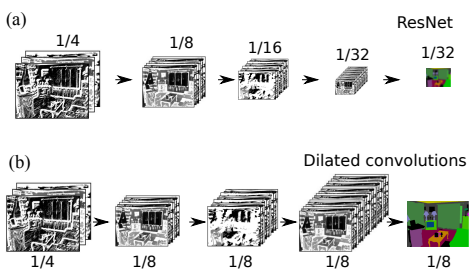Before moving further, a model that has shown high performance in prior to RefineNet Model is called as ResidualNet Model(ResNet in short). Since RefineNet is built on top of ResNet it is insightful to look at some of the base code for the fundamental functions that it is performing. The functions that the layer factory is required to perform are,

function name Description
batchnorm performs batch normalization on 2d
conv3x3 performs convolution with a kernel size of 3
conv1x1 performs convolution with a kernel size of 1
convbnrelu optionally creates a convolutional layer with or without ReLU

It also contains 2 classes,

class name Description
CRPBlock Its constructor uses conv3x3 to create and initialize a CRPBlock, along with a function forward which establishes the hops through maxpooling using n_stages
RCUBlock Its constructor uses conv3x3 to create and initialize a CRPBlock, along with a function forward which applies the relu activation to each stage in every block

The code for the same is,

``````import torch.nn as nn
import torch.nn.functional as F

def batchnorm(in_planes):
"batch norm 2d"
return nn.BatchNorm2d(in_planes, affine=True, eps=1e-5, momentum=0.1)

def conv3x3(in_planes, out_planes, stride=1, bias=False):
return nn.Conv2d(in_planes, out_planes, kernel_size=3, stride=stride,

def conv1x1(in_planes, out_planes, stride=1, bias=False):
"1x1 convolution"
return nn.Conv2d(in_planes, out_planes, kernel_size=1, stride=stride,

def convbnrelu(in_planes, out_planes, kernel_size, stride=1, groups=1, act=True):
"conv-batchnorm-relu"
if act:
return nn.Sequential(nn.Conv2d(in_planes, out_planes, kernel_size, stride=stride, padding=int(kernel_size / 2.), groups=groups, bias=False),
batchnorm(out_planes),
nn.ReLU6(inplace=True))
else:
return nn.Sequential(nn.Conv2d(in_planes, out_planes, kernel_size, stride=stride, padding=int(kernel_size / 2.), groups=groups, bias=False),
batchnorm(out_planes))

class CRPBlock(nn.Module):

def __init__(self, in_planes, out_planes, n_stages):
super(CRPBlock, self).__init__()
for i in range(n_stages):
setattr(self, '{}_{}'.format(i + 1, 'outvar_dimred'),
conv3x3(in_planes if (i == 0) else out_planes,
out_planes, stride=1,
bias=False))
self.stride = 1
self.n_stages = n_stages

def forward(self, x):
top = x
for i in range(self.n_stages):
top = self.maxpool(top)
top = getattr(self, '{}_{}'.format(i + 1, 'outvar_dimred'))(top)
x = top + x
return x

stages_suffixes = {0 : '_conv',
1 : '_conv_relu_varout_dimred'}

class RCUBlock(nn.Module):

def __init__(self, in_planes, out_planes, n_blocks, n_stages):
super(RCUBlock, self).__init__()
for i in range(n_blocks):
for j in range(n_stages):
setattr(self, '{}{}'.format(i + 1, stages_suffixes[j]),
conv3x3(in_planes if (i == 0) and (j == 0) else out_planes,
out_planes, stride=1,
bias=(j == 0)))
self.stride = 1
self.n_blocks = n_blocks
self.n_stages = n_stages

def forward(self, x):
for i in range(self.n_blocks):
residual = x
for j in range(self.n_stages):
x = F.relu(x)
x = getattr(self, '{}{}'.format(i + 1, stages_suffixes[j]))(x)
x += residual
return x

``````

Now ResNet models can be created with different variants using the above entities, the concepts required for understanding some of them are clearly explained in An Overview of ResNet and its Variants

## RefineNet Model

To overcome this drawback we look at a new hybrid model architecture that creatively derives from existing techniques and fuses them to find a more efficient solution to semantically segment with high resolution.

The refinement model comprises 2 core components,

### RefineNet blocks:

RefineNet blocks are 3-stage processing entities each handling and learning features of data on different levels of the model. The 3-stages of each block comprise the following components,

1. Adaptive Convolution - performs layer learning and processing on a level corresponding to down-sampled data using RCUs (Residual Convolutional Units).
2. Multi-Resolution Fusion - combines data from both short-range and long-range processed input streams.
3. Chained Resolution Pooling - recursively pools and convolves the combined data and maintains results in a chain manner using summation.

This can be visualized as,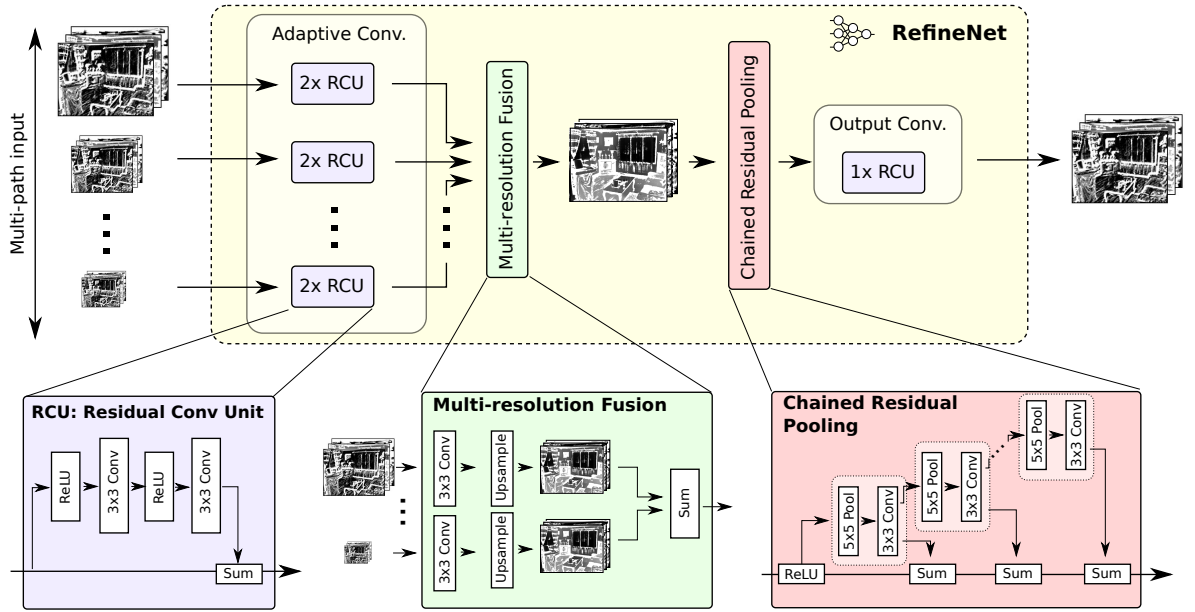### Multi-path Refinement

The use of residual connections with identity mappings enables gradients to be directly propagated within RefineNet Model via local residual connections, this also directly propagates to the input paths via long-range residual connections. In essence, each resolution level information is captured by using RefineNet blocks and with an interconnected architecture, styled in a hand-over fashion (using short and long connections) from which we are able to establish effective comprehension of the segments.

This can be visualized as,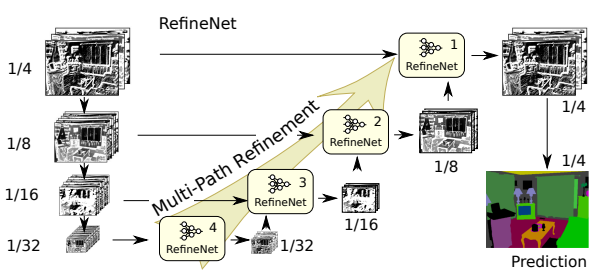It is obvious that this architecture can be modified in accordance with different datasets, a few typical modifications include,

1. Cascading - change in the number of RefineNet block layers
2. Scaling - change in the number of data scaled inputs. This is the addition of ResNet concept to the model

with clarity, the above-depicted model is a 4-cascaded RefineNet with 1-scale ResNet. Similarly, the below model is a 4-cascaded RefineNet with 2-scale ResNet.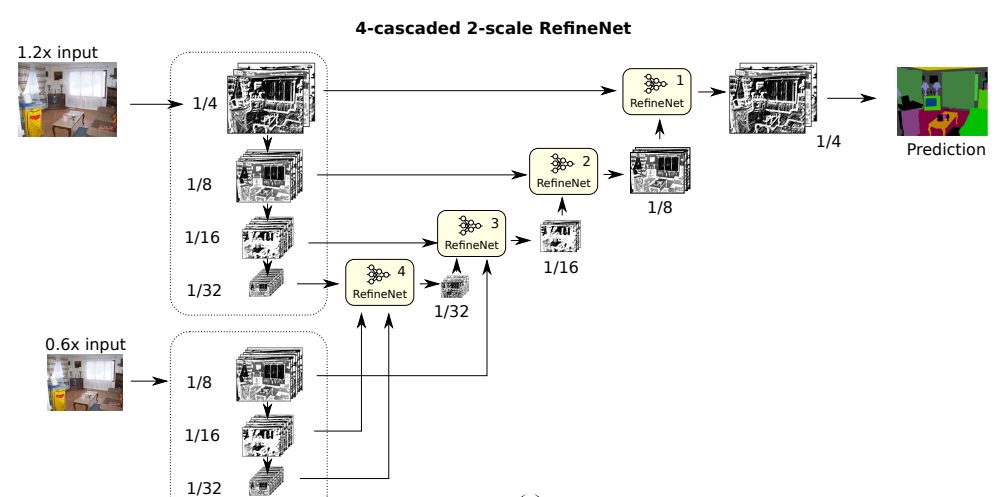This can be implemented in python using a class similar to the one shown below,

``````class RefineNet(nn.Module):

def __init__(self, block, layers, num_classes=21):
self.inplanes = 64
super(RefineNet, self).__init__()
self.do = nn.Dropout(p=0.5)
self.conv1 = nn.Conv2d(3, 64, kernel_size=7, stride=2, padding=3,
bias=False)
self.bn1 = nn.BatchNorm2d(64)
self.relu = nn.ReLU(inplace=True)
self.layer1 = self._make_layer(block, 64, layers)
self.layer2 = self._make_layer(block, 128, layers, stride=2)
self.layer3 = self._make_layer(block, 256, layers, stride=2)
self.layer4 = self._make_layer(block, 512, layers, stride=2)
self.p_ims1d2_outl1_dimred = conv3x3(2048, 512, bias=False)
self.adapt_stage1_b = self._make_rcu(512, 512, 2, 2)
self.mflow_conv_g1_pool = self._make_crp(512, 512, 4)
self.mflow_conv_g1_b = self._make_rcu(512, 512, 3, 2)
self.mflow_conv_g1_b3_joint_varout_dimred = conv3x3(512, 256, bias=False)
self.p_ims1d2_outl2_dimred = conv3x3(1024, 256, bias=False)
self.adapt_stage2_b = self._make_rcu(256, 256, 2, 2)
self.mflow_conv_g2_pool = self._make_crp(256, 256, 4)
self.mflow_conv_g2_b = self._make_rcu(256, 256, 3, 2)
self.mflow_conv_g2_b3_joint_varout_dimred = conv3x3(256, 256, bias=False)

self.p_ims1d2_outl3_dimred = conv3x3(512, 256, bias=False)
self.adapt_stage3_b = self._make_rcu(256, 256, 2, 2)
self.mflow_conv_g3_pool = self._make_crp(256, 256, 4)
self.mflow_conv_g3_b = self._make_rcu(256, 256, 3, 2)
self.mflow_conv_g3_b3_joint_varout_dimred = conv3x3(256, 256, bias=False)

self.p_ims1d2_outl4_dimred = conv3x3(256, 256, bias=False)
self.adapt_stage4_b = self._make_rcu(256, 256, 2, 2)
self.mflow_conv_g4_pool = self._make_crp(256, 256, 4)
self.mflow_conv_g4_b = self._make_rcu(256, 256, 3, 2)

self.clf_conv = nn.Conv2d(256, num_classes, kernel_size=3, stride=1,

def _make_crp(self, in_planes, out_planes, stages):
layers = [CRPBlock(in_planes, out_planes,stages)]
return nn.Sequential(*layers)

def _make_rcu(self, in_planes, out_planes, blocks, stages):
layers = [RCUBlock(in_planes, out_planes, blocks, stages)]
return nn.Sequential(*layers)

def _make_layer(self, block, planes, blocks, stride=1):
downsample = None
if stride != 1 or self.inplanes != planes * block.expansion:
downsample = nn.Sequential(
nn.Conv2d(self.inplanes, planes * block.expansion,
kernel_size=1, stride=stride, bias=False),
nn.BatchNorm2d(planes * block.expansion),
)

layers = []
layers.append(block(self.inplanes, planes, stride, downsample))
self.inplanes = planes * block.expansion
for i in range(1, blocks):
layers.append(block(self.inplanes, planes))

return nn.Sequential(*layers
``````

In addition to this, the inclusion of the forward function and a function that derives the model from another class that defines the bottleneck of the model will constitute the essential parts of a RefineNet variant.

The concept of level-specific information exchange and processing is constructed for a 4 level model using 4 layers in the code. By striking the similarity of the functions used in the codes for ResNet and RefineNet it is evident that RefineNet is created based on ResNet.

## Performance Comparison

This model has been extensively tested on multiple datasets, we are going to look at the results of different models on a practically implementable dataset like Cityscapes.

Cityscapes dataset consists of 2975 training images and 500 validation images, belonging to 19 classes. This dataset provides fine-grained pixel-level annotations of objects themed on street scene images from 50 different European cities. The results of the application of RefineNet model on Cityscapes dataset yield,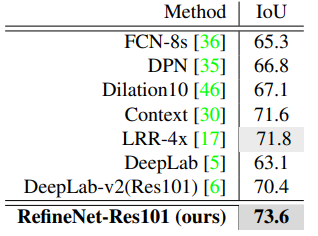The comparison between the actual and predicted segments is shown for a few sample instances,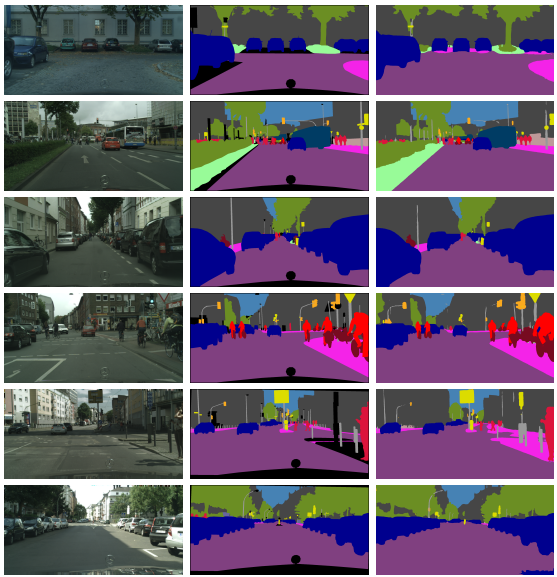### Advantages of the RefineNet Model are,

1. Creation Ease, can be trained end-to-end.
2. Flexible, can be cascaded and modified in various ways
3. Performance, produces better results on high-resolution images

### Disadvantages of the RefineNet Model are,

1. Model complexity
2. High processing, as the model is trained end-to-end it needs considerable resources to implement.

## Applications

The application of Semantic Segmentation is broad due to its significance. Some domains where one can find use from it are,

1. Computer/Machine Vision
2. Healthcare and Medical
3. Sports
4. Automobile
5. Security and Defence

Most of this article was inspired by the professional presentation and performance enhancements achieved by RefineNet: Multi-Path Refinement Networks for High-Resolution Semantic Segmentation

 Lin, G., Milan, A., Shen, C. and Reid, I., 2017. Refinenet: Multi-path refinement networks for high-resolution semantic segmentation. In Proceedings of the IEEE conference on computer vision and pattern recognition (pp. 1925-1934).

The code for the complete RefineNet Model can be found here (by Guosheng Lin).#### B E Pranav Kumaar

B E Pranav Kumaar is a Machine Learning Developer, Intern at OpenGenus and is pursuing B. Tech in Computer Science Engineering and AI from Amrita Vishwa Vidyapeetham (2020 to 2024).